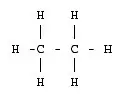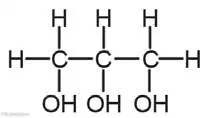# 3 Marks Question

1. Name the compound having the given structural formula. Which compound is formed when one hydrogen atom from each carbon is replaced by -OH group and also, write its one application.2. The compound having the given structural formula is Propane.
Glycerol is formed when one hydrogen atom from each carbon is replaced by -OH group. It is used in a stamp pad.
3. Which compound is represented by general formula CnH2n+1OH? If value of n is 2 in this formula which compound is formed? Write its structural formula. If OH of this compound is replaced by H which compound is formed? Write its name and molecular formula.
4. Alcohol is represented by general formula CnH2n+1OH.

If the value of n is 2 in this formula the compound formed is ethyl alcohol. Its structural formula is:If OH of this compound is replaced by H Ethane compound is formed, its molecular formula is C2H6.

5. Write down the general formula of alkyne. Write the name and structural formula of the member of alkyne which contains an equal number of hydrogen and carbon. If such a compound is reacted with lots of hydrogen what happens?
6. The general formula of alkyne is CnH2n-2.
The name of the member of alkyne which contains an equal number of hydrogen and carbon is ethyne and its structural formula is:
H – C ≡ C – H
If such compound in reacted with lots of hydrogen ethane (C2H6) is formed.
7. Observe the following structural formula and answer the given questions.1. Write the name and molecular formula of the hydrocarbon given in the figure.
2. Trihydric Alcohol or Glycerol and molecular formula is C3H5(OH)3.
3. Why is it called trihydric alcohol?
4. It has three OH group so, it is called trihydric alcohol.
5. Mention any one practical applications of this hydrocarbon.
6. It is used in fruits and food materials as preservatives.